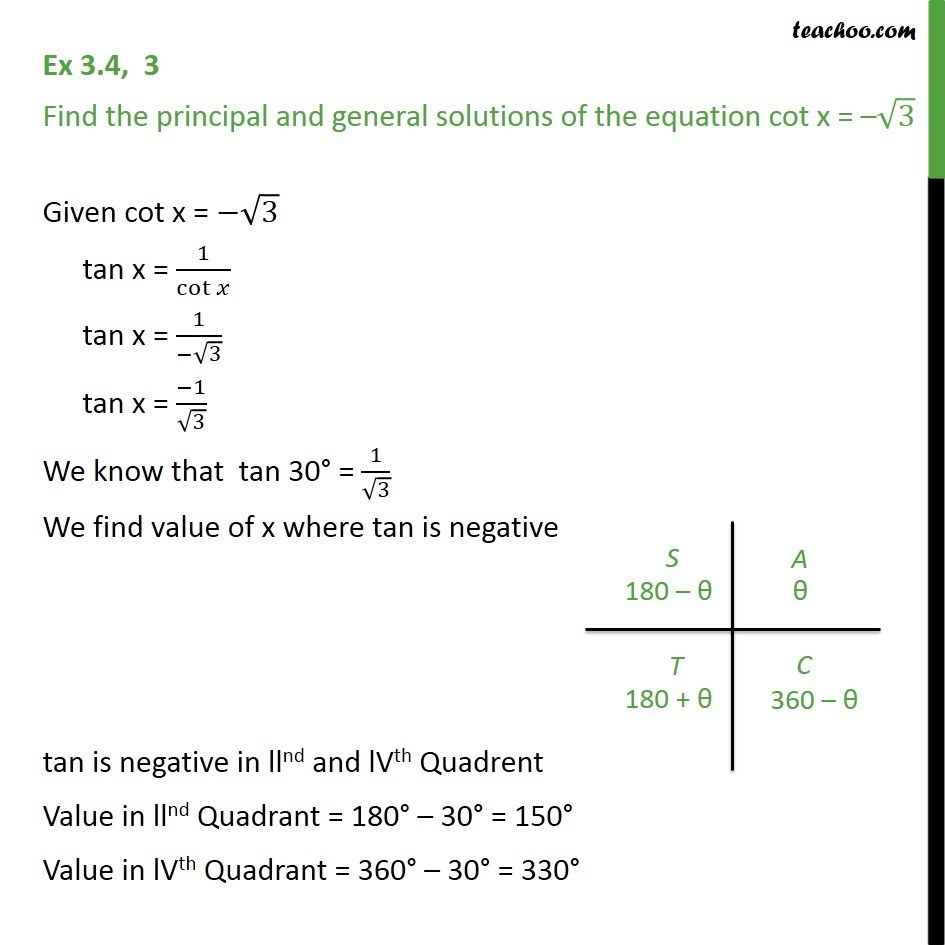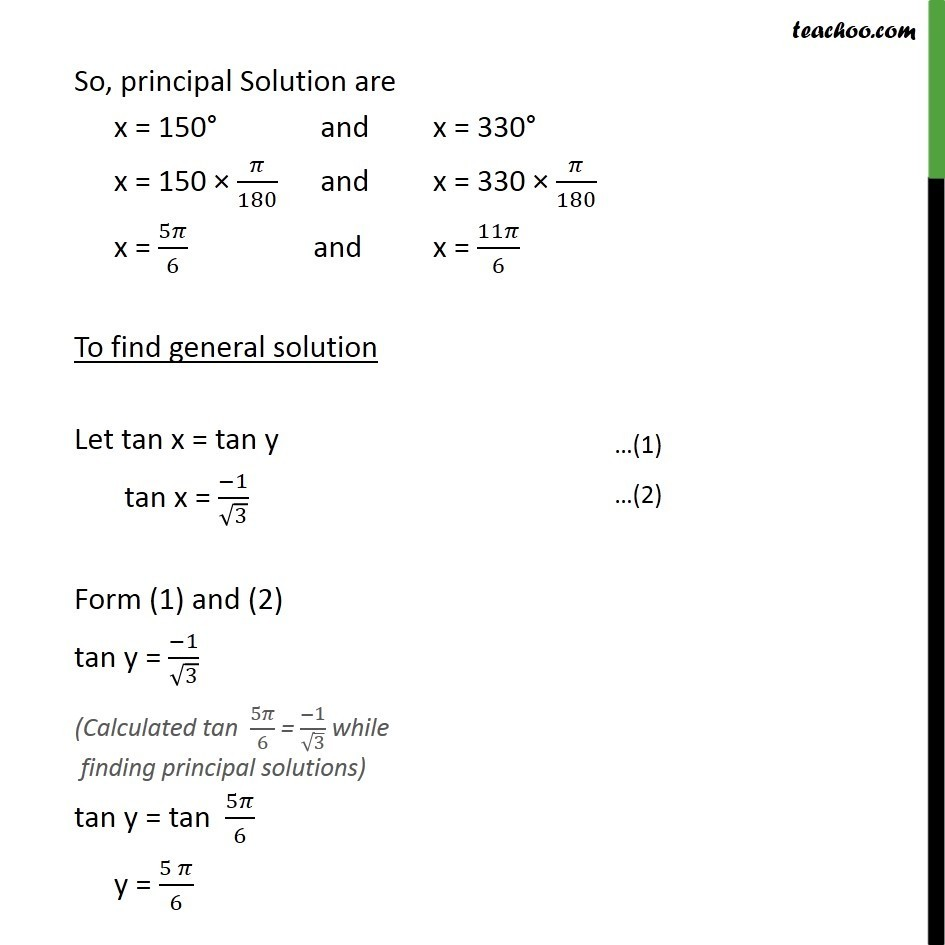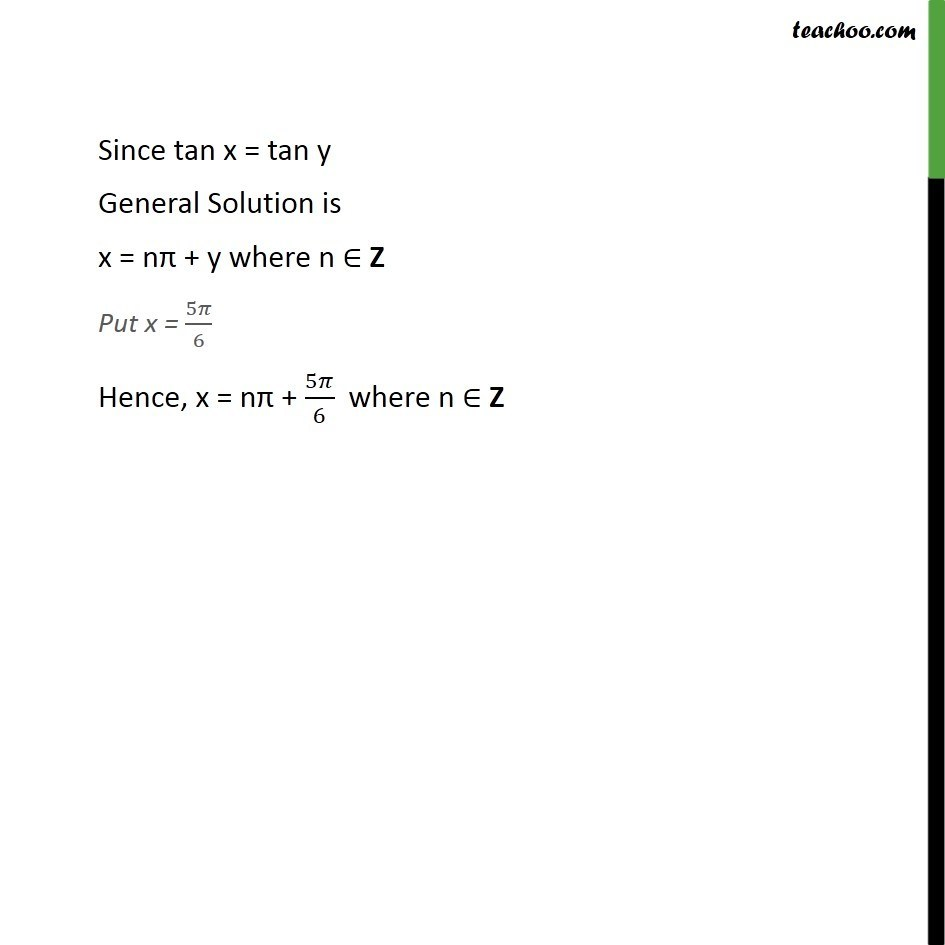1. Chapter 3 Class 11 Trigonometric Functions
2. Serial order wise
3. Ex 3.4

Transcript

Ex 3.4, 3 Find the principal and general solutions of the equation cot x = –√3 Given cot x = −√3 tan x = 1/cot⁡𝑥 tan x = 1/(−√3) tan x = (−1)/√3 We know that tan 30° = 1/√3 We find value of x where tan is negative tan is negative in llnd and lVth Quadrent Value in llnd Quadrant = 180° – 30° = 150° Value in lVth Quadrant = 360° – 30° = 330° So, principal Solution are x = 150° and x = 330° x = 150 × 𝜋/180 and x = 330 × 𝜋/180 x = 5𝜋/6 and x = 11𝜋/6 To find general solution Let tan x = tan y tan x = (−1)/√3 Form (1) and (2) tan y = (−1)/√3 tan y = tan 5𝜋/6 y = (5 𝜋)/6 Since tan x = tan y General Solution is x = nπ + y where n ∈ Z Put x = 5𝜋/6 Hence, x = nπ + 5𝜋/6 where n ∈ Z

Ex 3.4

About the AuthorDavneet Singh
Davneet Singh is a graduate from Indian Institute of Technology, Kanpur. He has been teaching from the past 9 years. He provides courses for Maths and Science at Teachoo.